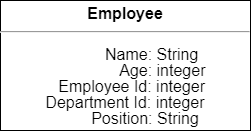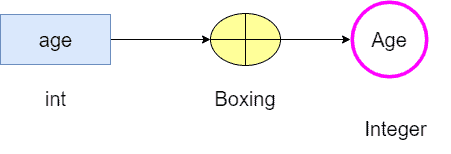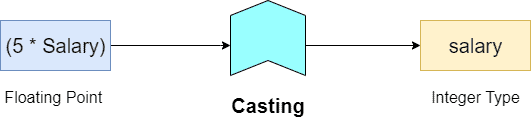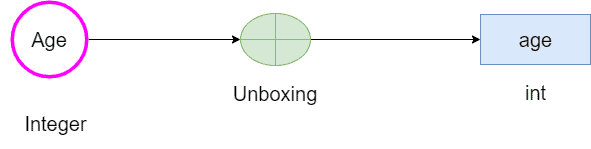## 1. Introduction

In this tutorial, we’ll learn boxing and unboxing operation in computer programming.

## 2. Datatypes

We usually have three main categories of datatypes:

1. Native datatype
2. Wrapper datatype
3. User-defined datatype

Let’s first recap some concepts about each datatype in the following subsections.

### 2.1. Native Datatypes

Every programming language has some native datatypes that are provided in its core. We call them primitive data types. The system maps a primitive datatype directly at the lowest level of the language implementation (machine level). Further, primitives are immutable (we can’t change their type) and scalar.

For example, in Java language, we have int as a primitive datatype to represent fixed point values (integer values). In such a case, the underlying machine architecture determines the datatype size. In particular, the int datatype is usually of size 4 bytes (32 bits).

The native datatypes category encompasses primitive types (e.g., signed and unsigned int, float, special type bool, data structs, and statically allocated arrays of basic types.

### 2.2. Wrapper Datatypes

Many programming languages, such as Java, provide wrapper classes to store native or primitive datatypes. We call these datatypes of wrapper ones.

For example, Java uses the Integer datatype for wrapping the native datatype int.

Wrapper datatypes provide extra functions (e.g., conversion to string) on the underlying primitive data type. Additionally, they wrap values of primitive types into objects to make parameter passing easier.

### 2.3. User Datatypes

A User-Defined Datatype (UDT) is a datatype that we construct from existing datatypes. So, we use a UDT to extend a native datatype or to create our customized datatype. For example, we can use a class to templatize an employee record:## 3. Boxing

With the necessary background reviewed, we now study the boxing operation.

### 3.1. Concept

From a computer programming perspective, we can understand boxing as a wrapping operation to transform a primitive type into an object (wrapper datatype).

Boxing typically uses object pointers to store value on the heap. Once we box a datatype, we get an object. This object uses more memory and is relatively complex. After that, the system stores this object on the heap. Hence, we consume more memory and have increased lookup time.

Why increased lookup time? Because we take two memory lookups to access the boxed value. Our first access takes us to the boxed object pointer, and the second takes us to the primitive value. So, using boxed values tends to slow the execution of a software program.

### 3.2. Example

Let’s understand the boxing operation with an example.

Let’s say we use the variable age to store the age of an employee. Hence, the age value is a primitive integer number (int in Java). Then, we can box it to an object of a particular type (Integer in Java):Thus, the boxed datatype provides multiple functions to operate the primitive value. Among them, we can cite the conversion of the value to a string. So we can print it on the screen.

Many compilers execute autoboxing as an optimization measure. So we don’t have to explicitly convert conversion a primitive datatype to its equivalent wrapper type. In such a way, we not only reduce our code footprint but also reduce the complexity.

Furthermore, boxed values have additional metadata and structural organizations that our primitive datatypes don’t have: since they are objects, they can have several fields, methods, and behaviors.

### 3.4. Boxing vs. Casting

Both boxing and casting have similar functionality, but we can find a subtle difference: we commonly execute boxing operations on a datatype, whereas we execute casting on an expression.

So, in casting, we explicitly convert an expression result to a given type. However, it can result in loss of information because of conversion from a higher precision type to a lower precision type.

As an example, we can cast on an expression involving the variable Salary of floating point type to get another variable, salary for fixed point (integer) type. So, if the variable Salary holds 1000.5, then after casting, our new variable age will hold 5002 (and not 5002.5).## 4. Unboxing

Now, let’s understand the unboxing operation!

### 4.1. Concept

Unboxing is the reverse transformation of boxing. So, we extract the primitive value of an object from its wrapper object.

### 4.2. Example

We saw a boxing operation in the example of Section 3.2. Here, we can unbox the object Age back to our primitive type int:## 5. Conclusion

In this article, we studied boxing and unboxing data in the realm of computer programming.

Boxing is the process of converting a primitive datatype into an object wrapper datatype, and unboxing is the process of converting a value from an object wrapper type back to the native primitive value. Further on, we can say that both boxing and unboxing operations are a subset of typecasting.

We can conclude that boxing and unboxing can make our programs slower and more memory intensive when compared to using native datatypes. But they give us additional capabilities and make our code more robust and flexible.